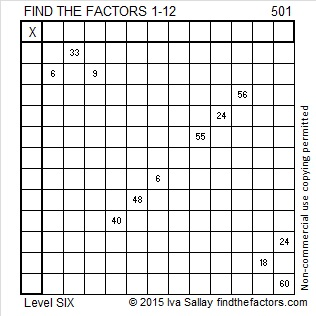# 501 and Level 6

2 + 3 + 5 + 7 + 11 + 13 + 17 + 19 + 23 + 29 + 31 + 37 + 41 + 43 + 47 + 53 + 59 + 61 = 501. That was the first 18 prime numbers.Print the puzzles or type the solution on this excel file: 12 Factors 2015-05-18

—————————————————————————————————

• 501 is a composite number.
• Prime factorization: 501 = 3 x 167
• The exponents in the prime factorization are 1 and 1. Adding one to each and multiplying we get (1 + 1)(1 + 1) = 2 x 2 = 4. Therefore 501 has exactly 4 factors.
• Factors of 501: 1, 3, 167, 501
• Factor pairs: 501 = 1 x 501 or 3 x 167
• 501 has no square factors that allow its square root to be simplified. √501 ≈ 22.383029—————————————————————————————————This site uses Akismet to reduce spam. Learn how your comment data is processed.